Structure Based or Whitebox Testing Techniques | HCLTech

# Structure Based or Whitebox Testing Techniques

View Tags

## Structure Based or Whitebox Testing Techniques February 26, 2015

There are various types of software testing techniques. Each individual technique is good at finding a particular type of defect. Each testing technique falls into a number of different categories.

There are two main categories - static and dynamic. Dynamic techniques are subdivided into three more categories:

• Specification-based Testing (black-box, also known as behavioral techniques)
• Structure-based Testing (white-box or structural techniques)
• Experience-based Testing

Static Techniques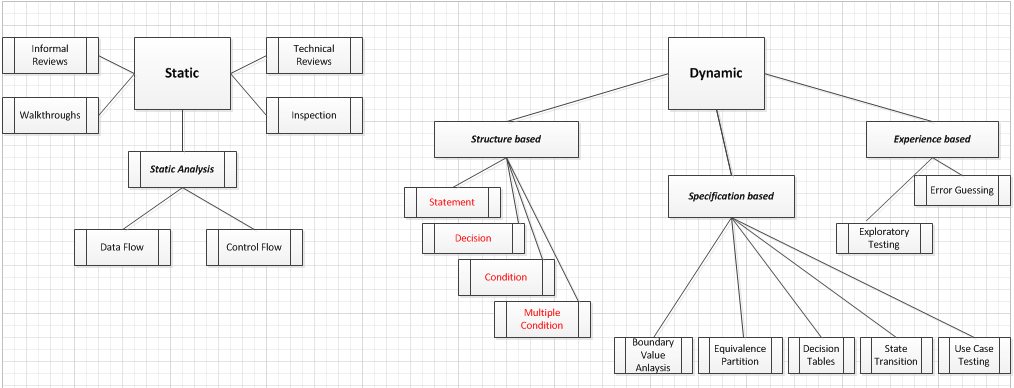Figure 1: Static Techniques

Dynamic TechniquesFigure 2: Dynamic Techniques

Let’s discuss structure-based testing marked in red in the above diagram.

Structure-based testing techniques use the internal structure of a software to derive test cases. They are commonly called 'white-box' or 'glass-box' techniques. Structure-based techniques can also be used at all levels of testing.

E.g.: In component testing, component integration testing, and system and acceptance testing.

Structure-based test design techniques are a good way to help ensure more breadth of testing. To measure what percentage of code has been exercised by a test suite, one or more coverage criteria is used. A coverage criterion is usually defined as a rule or requirement, which a test suite needs to satisfy.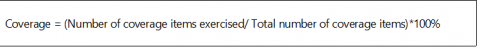There are a number of coverage criteria. Let’s discuss Statement, Decision (Branch) and Path coverage, and understand how to calculate, with examples.

For e.g.:

IF X+Y > 100 THEN

Print “Large”

ENDIF

If X > 50 THEN

Print “X Large”

ENDIF

For calculating Statement, Decision (Branch) and Path coverage, I’ve created a flow chart for better understanding: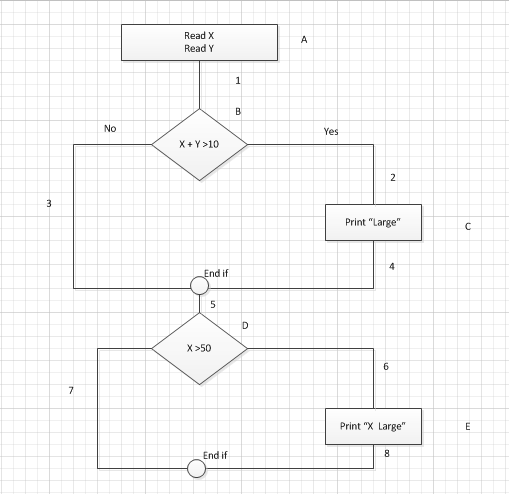Figure 3: Coverage - Flow Chart

Reference:

• Nodes (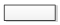,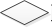) represent statement of code [E.g.: entry, exit, decisions]
• Edges (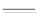) represent links between nodes

Statement Coverage

Statement coverage is a whitebox testing technique technique where the all the statements at the source code are executed at least once. To calculate Statement Coverage, find out the shortest number of paths following which all the nodes will be covered.

In the above example, in case of “Yes”, while traversing through each statement of code and the traversing path (A1-B2-C4-5-D6-E8), all the nodes are covered. So by traveling through only one path all the nodes (A, B, C, D and E) are covered.

Statement coverage (SC) =1

Branch/Decision Coverage

Branch coverage covers both ways (true and false). It covers all the possible outcomes of each condition at least once. Branch coverage is a whitebox testing method that ensures that every possible branch from each decision point in the code is executed at least once. To calculate Branch coverage, find out the minimum number of paths which ensure covering of all the edges.

In the above example, in case of traversing through a ‘Yes’ decision, path (A1-B2-C4-5-D6-E8), maximum numbers of edges (1, 2, 4, 5, 6 and 8) are covered but edges 3 and 7 are left out. To cover these edges, we have to follow (A1-B3-5-D7). So by travelling through two paths (Yes, No), all the edges (1, 2, 3, 4, 5, 6, 7, 8) are covered.

Branch Coverage /Decision Coverage (BC) = 2

Path Coverage

It is executed in such a way that every path is executed at least once. It ensures that every statement in the program is guaranteed to be executed at least one time. Path Coverage ensures covering all the paths from beginning to end, in the above example. All the possible paths are:

A1-B3-5-D7
A1-B2-C4-5-D6-E8
A1-B2-C4-5-D7
A1-B3-5-D6-E8
Path coverage (PC) = 4

Condition Coverage

It is related to decision coverage but has better sensitivity to the control flow. Condition coverage reports the true or false outcome of each condition. It measures the conditions independently of each other. Multiple condition coverage is also known as condition combination coverage.

Let us take an example to explain condition coverage:

IF ("X && Y")

In order to suffice valid condition coverage for this pseudo-code, the following tests will be sufficient.

TEST 1: X=TRUE, Y=FALSE

TEST 2: X=FALSE, Y=TRUE

I hope this blog has helped you understand and calculate the coverage in White Box Code testing.

Reference

http://www.istqb.guru/how-to-calculate-statement-branchdecision-and-path-coverage-for-istqb-exam-purpose/